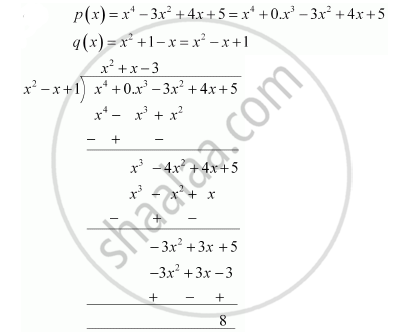# Divide the polynomial p(x) by the polynomial g(x) and find the quotient and remainder in each of the following :  p(x) = x^4 – 3x^2 + 4x + 5,   g(x) = x^2 + 1 – x - Mathematics

Divide the polynomial p(x) by the polynomial g(x) and find the quotient and remainder in each of the following : p(x) = x4 – 3x2 + 4x + 5,   g(x) = x2 + 1 – x

#### SolutionQuotient = x2 + x - 3

Remainder = 8

Concept: Division Algorithm for Polynomials
Is there an error in this question or solution?
Chapter 2: Polynomials - Exercise 2.3 [Page 36]

#### APPEARS IN

NCERT Class 10 Maths
Chapter 2 Polynomials
Exercise 2.3 | Q 1.2 | Page 36

Share